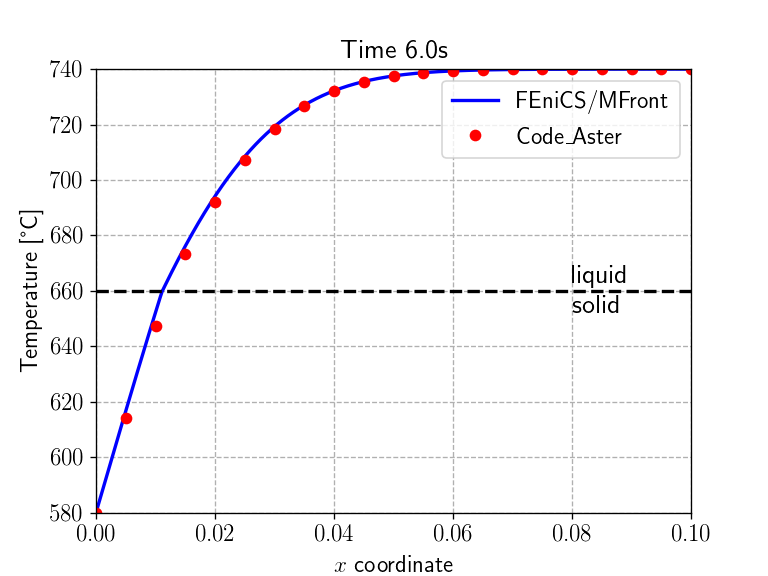true

In this demo, we expand on the stationnary nonlinear heat transfer demo and consider a transient heat equation with non-linear heat transfer law including solid/liquid phase change. This demo corresponds to the TTNL02 elementary test case of the code_aster finite-element software.

Source files:

# Transient heat equation using an enthalpy formulation

The transient heat equation writes:

$\rho C_p \dfrac{\partial T}{\partial t} = r-\operatorname{div}\mathbf{j}$

where $$\rho$$ is the material density, $$C_p$$ the heat capacity (at constant pressure) per unit of mass, $$r$$ represents heat sources and $$\mathbf{j}$$ is the heat flux.

In the case of phase changes, the heat capacity exhibits large discontinuities near the transition temperature. It is therefore more suitable to work with the enthalpy density defined as:

$h(T) = \int_{T_0}^{T} \rho C_p dT$

yielding the following heat equation: $\dfrac{\partial h}{\partial t} = r-\operatorname{div}\mathbf{j}$

# Description of the non-linear heat transfer law with phase change

The thermal material is described by the following non linear Fourier Law:

$\mathbf{j}=-k\left(T\right)\,\mathbf{\nabla} T$

where the thermal conductivity $$k$$ is initially assumed to be given by:

$k\left(T\right)=\begin{cases} k_s & \text{if }T < T_m \\ k_l & \text{if }T > T_m \end{cases}$ where $$k_s$$ (resp. $$k_l$$) denotes the solid (resp. liquid) phase conductivity and $$T_m$$ is the solid/liquid transition temperature.

The enthalpy is assumed to be given by:

$h\left(T\right)=\begin{cases} c_sT & \text{if }T < T_m \\ c_l(T-T_m)+c_sT_m+\Delta h_{s/l} & \text{if }T > T_m \end{cases}$

where $$c_s=\rho_sC_{p,s}$$ (resp. $$c_l=\rho_lC_{p,l}$$) is the volumic heat capacity of the solid (resp. liquid) phase. It can be observed that the enthalpy exhibits a discontinuity at the phase transition equal to $$\Delta h_{s/l}$$ which represents the latent heat of fusion per unit volume.# A smoothed version

The enthalpy discontinuity $$\Delta h_{s/l}$$ poses convergence difficulties for the Newton resolution. A classical remedy consists in considering a smoothed version of the previous law, such as:

$k\left(T\right)=\begin{cases} k_s & \text{if }T < T_s \\ k_s + (k_l-k_s)\dfrac{T-T_s}{T_\text{smooth}} & \text{if } T_s \leq T \leq T_l\\ k_l & \text{if }T > T_l \end{cases}$ and $h\left(T\right)=\begin{cases} c_sT & \text{if }T < T_s \\ c_sT_s+\left(\dfrac{cs+cl}{2}+\dfrac{\Delta h_{s/l}}{T_\text{smooth}}\right)(T-T_s) & \text{if } T_s \leq T \leq T_l \\ c_l(T-T_l)+c_sT_s+\dfrac{cs+cl}{2}T_\text{smooth}+\Delta h_{s/l} & \text{if }T > T_l \end{cases}$ where $$T_{smooth}=T_l-T_s$$ is a small transition temperature interval between $$T_s=T_m-T_\text{smooth}/2$$ the solidus temperature and $$T_l=T_m+T_\text{smooth}/2$$ the liquidus temperature.

# MFront implementation

## Gradient, flux and tangent operator blocks

Similarly to the stationnary nonlinear heat transfer demo, the MFront implementation relies on the DefaultGenericBehaviour DSL and declares the pair of temperature gradient and heat flux. In addition, the volumic enthalpy $$h$$ is also declared as an internal state variable. In addition to the two tangent operator blocks ∂j∕∂Δ∇T and ∂j∕∂ΔT already discussed in the first demo, we also declare the additional block ∂h∕∂ΔT, referring to the fact that the enthalpy will vary with the temperature and will enter the transient heat equation.

@DSL DefaultGenericBehaviour;
@Behaviour HeatTransferPhaseChange;
@Author Thomas Helfer / Jérémy Bleyer;
@Date 15 / 02 / 2019;

@Flux HeatFlux j;
j.setGlossaryName("HeatFlux");

@StateVariable real h;
h.setEntryName("Enthalpy"); //per unit of volume

@AdditionalTangentOperatorBlock ∂h∕∂ΔT;

## Material parameters and local variables

We now declare the various material properties corresponding to those of aluminium. The material parameters are assumed to be uniform for both phases. Finally, we also introduce the smoothing temperature width $$T_\text{smooth}$$.

@Parameter Tₘ = 933.15;        // [K]
Tₘ.setEntryName("MeltingTemperature");
@Parameter kₛ = 210;           // [W/m/K]
kₛ.setEntryName("SolidConductivity");
@Parameter cₛ = 3.e6;          // [J/m^3/K]
cₛ.setEntryName("SolidHeatCapacity");
@Parameter kₗ = 95;            // [W/m/K]
kₗ.setEntryName("LiquidConductivity");
@Parameter cₗ = 2.58e6;        // [J/m^3/K]
cₗ.setEntryName("LiquidHeatCapacity");
@Parameter Δhₛₗ = 1.08048e9;   // [J/m^3]
Δhₛₗ.setEntryName("FusionEnthalpy");
@Parameter Tₛₘₒₒₜₕ = 0.1;      // smoothing temperature width [K]
Tₛₘₒₒₜₕ.setEntryName("Tsmooth");

We define some local variables corresponding to the values of the conductivity $$k$$, the volumic heat capacity $$c$$ and the derivative of the heat conductivity with respect to the temperature.

@LocalVariable thermalconductivity k;
@LocalVariable real c;
@LocalVariable real ∂k∕∂T;

## Integration of the behaviour

Again, the behaviour integration is straightforward: after computing the temperature at the end of the time step T_, we compute the thermal conductivity, its derivative with respect to the temperature, the volumic enthalpy and the volumic heat capacity depending on whether T_ belongs to the solid state ($$T\leq T_s$$), the liquid state ($$T\geq T_l$$) or to the transition region ($$T_s \leq T \leq T_l$$). We finish by computing the heat flux.

@Integrator {
const auto T_ = T + ΔT;     // current temperature
const auto Tₛ = Tₘ-Tₛₘₒₒₜₕ/2; // solidus temperature
const auto Tₗ = Tₘ+Tₛₘₒₒₜₕ/2; // liquidus temperature
if(T_<Tₛ){                  // solid state
k = kₛ;
c = cₛ;
h = cₛ*T_;
∂k∕∂T = 0.;
} else if (T_ > Tₗ) {        // liquid state
k = kₗ;
c = cₗ;
h = cₗ*(T_-Tₗ)+Δhₛₗ+cₛ*Tₛ+(cₛ+cₗ)*Tₛₘₒₒₜₕ/2;
∂k∕∂T = 0.;
} else {                    // solid/liquid smooth transition
k = kₛ + (kₗ-kₛ)*(T_-Tₛ)/Tₛₘₒₒₜₕ;
h = cₛ*Tₛ+((cₛ+cₗ)/2+Δhₛₗ/Tₛₘₒₒₜₕ)*(T_-Tₛ);
c = Δhₛₗ/(Tₗ-Tₛ);
∂k∕∂T = -(kₗ-kₛ)/Tₛₘₒₒₜₕ;
}
// heat flux
j = -k ⋅ (∇T + Δ∇T);
}  // end of @Integrator

# Tangent operator

The computation of the tangent operator blocks is then straightforward:

@TangentOperator {
∂j∕∂Δ∇T = -k * tmatrix<N, N, real>::Id();
∂j∕∂ΔT = ∂k∕∂T * (∇T + Δ∇T);
∂h∕∂ΔT = c;
}  // end of @TangentOperator

# FEniCS implementation

## Geometry and material

We consider a rectanglar domain of length 0.1 with imposed temperatures T0 (resp. Ti) on the left (resp. right) boundaries. We look here for the temperature field T using a $$P^2$$-interpolation which is initially at the uniform temperature Ti.

%matplotlib notebook
import matplotlib.pyplot as plt
from dolfin import *
import mgis.fenics as mf
import numpy as np

length = 0.1
width = 0.01
Nx = 1000
Ny = 5
mesh = RectangleMesh(Point(0., 0.), Point(length, width), Nx, Ny, "crossed")
x = np.linspace(0, length, Nx)

V = FunctionSpace(mesh, "CG", 2)
T = Function(V, name="Temperature")

def left(x, on_boundary):
return near(x, 0) and on_boundary
def right(x, on_boundary):
return near(x, length) and on_boundary

T0 = Constant(853.15)
Ti = Constant(1013.15)
T.interpolate(Ti)

bc = [DirichletBC(V, T0, left),
DirichletBC(V, Ti, right)]

We now load the material behaviour HeatTransferPhaseChange and also change the default value of Tsmooth to a slightly larger one (but still sufficiently small). Note that the mesh must be sufficiently refined to use a smaller value. Indeed, the spatial resolution must be able to capture with a few elements the sharp transition which will occur during the phase change. We also verify that 3 different tangent blocks have indeed been defined, the last one involving the internal state variable Enthalpy with respect to the temperature.

material = mf.MFrontNonlinearMaterial("./src/libBehaviour.so",
"HeatTransferPhaseChange",
hypothesis="plane_strain",
parameters={"Tsmooth": 1.})
print(["d{}_d{}".format(*t) for t in material.get_tangent_block_names()])
['dHeatFlux_dTemperatureGradient', 'dHeatFlux_dTemperature', 'dEnthalpy_dTemperature']

## Time discretization of the heat equation

The heat equation must also be discretized in time. We use here the $$\theta$$-method and approximate:

$\left.\dfrac{\partial h}{\partial t}\right|_{t=t_{n+\theta}} \approx \dfrac{h_{t=t_{n+1}}-h_{t=t_{n}}}{\Delta t} = r_{t=t_{n+\theta}}-\operatorname{div}\mathbf{j}_{t=t_{n+\theta}}$

where $$\star_{t=t_{n+\theta}}= \theta\star_{t=t_{n+1}}+(1-\theta)\star_{t=t_{n}}$$.

The weak formulation therefore reads (in the absence of source terms):

Find $$T\in V$$ such that: $\int_\Omega \left((h_{t=t_{n+1}}(T)-h_{t=t_{n}})\widehat{T} - \Delta t (\theta\mathbf{j}_{t=t_{n+1}}(T, \nabla T)+(1-\theta)\mathbf{j}_{t=t_{n}})\cdot \nabla \widehat{T} \right)\text{ dx} = 0$

in which, at time $$t_{n+1}$$, both the enthalpy $$h_{t=t_{n+1}}$$ and the heat flux $$\mathbf{j}_{t=t_{n+1}}$$ are non-linear functions of the unknown temperature.

## Problem formulation

We therefore see that the above non-linear problem does not fit into the default form of a MFrontNonlinearProblem residual. We will therefore have to specify its form manually. To do so, we need to get the functions h and j associated to the current values of the enthalpy and the heat flux.

Second, we must call the initialize method which initializes the functions associated with gradients, fluxes, external and internal state variables objects and the corresponding tangent blocks. All gradients and external state variables must have been registered before calling this method. In this case, we rely on the automatic registration of the temperature and its gradient.

Finally, to implement the $$\theta$$ time discretization scheme, we will also need to keep track of the enthalpy and heat flux values at the previous time step. We can simply define these new functions as deep copies of h and j. Doing so, h_old and j_old will also be Quadrature functions.

problem = mf.MFrontNonlinearProblem(T, material, quadrature_degree=2, bcs=bc)
h = problem.get_state_variable("Enthalpy")
j = problem.get_flux("HeatFlux")
problem.initialize()

j_old = j.copy(deepcopy=True)
h_old = h.copy(deepcopy=True)
    Automatic registration of 'TemperatureGradient' as grad(Temperature).

Automatic registration of 'Temperature' as an external state variable.

## Residual definition and tangent form computation

We are now ready to define the expression of the above residual. Note that we must use the integration measure dx associated with the MFrontNonlinearProblem containing the correct quadrature degree matching that of the various Quadrature functions. Finally, the tangent form can be automatically computed using the compute_tangent_form method from the residual expression and the structure of the different tangent blocks.

dt = Constant(0.)
theta = Constant(1.)
T_ = TestFunction(V)
j_theta = theta*j + (1-theta)*j_old
problem.residual = (T_*(h - h_old)-dt*dot(grad(T_), j_theta))*problem.dx
problem.compute_tangent_form()

## Time-stepping loop and comparison with code_aster results

We now implement the time-stepping loop which simply solves the non-linear problem and update the fields corresponding to the values at the previous time step. We also load the values of the one-dimensional temperature field $$T(x, t)$$ given in the code_aster test-case and compare them with what we obtain every second.

cA_results = np.loadtxt("results_code_Aster.csv", delimiter=",")
code_Aster_times = np.arange(1, 7)
Nsteps = 60
times = np.linspace(0, 6., Nsteps+1)
for (t, delta_t) in zip(times[1:], np.diff(times)):
dt.assign(Constant(delta_t))

problem.solve(T.vector())

h_old.assign(h) # update enthalpy
j_old.assign(j) # update heat flux

sol_time = np.isclose(t, code_Aster_times)
if any(sol_time):
plt.figure()
plt.title("Time {:0.1f}s".format(t), fontsize=16)
ax1 = plt.gca()
ax1.set_xlabel('$x$ coordinate')
ax1.set_ylabel('Temperature [°C]')
ax1.plot(x, np.array([T(xi, width/2)-273.15 for xi in x]), "-b", label="FEniCS/MFront")
ax1.plot(cA_results[:, 0], cA_results[:, np.where(sol_time)+1], "or", label="Code\_Aster")
Tm = material.get_parameter("MeltingTemperature") - 273.15
ax1.plot(x, Tm + 0*x, "--k")
ax1.annotate("liquid\nsolid", xy=(0.08, Tm), fontsize=16, va="center")
plt.legend()
plt.show()
    <IPython.core.display.Javascript object>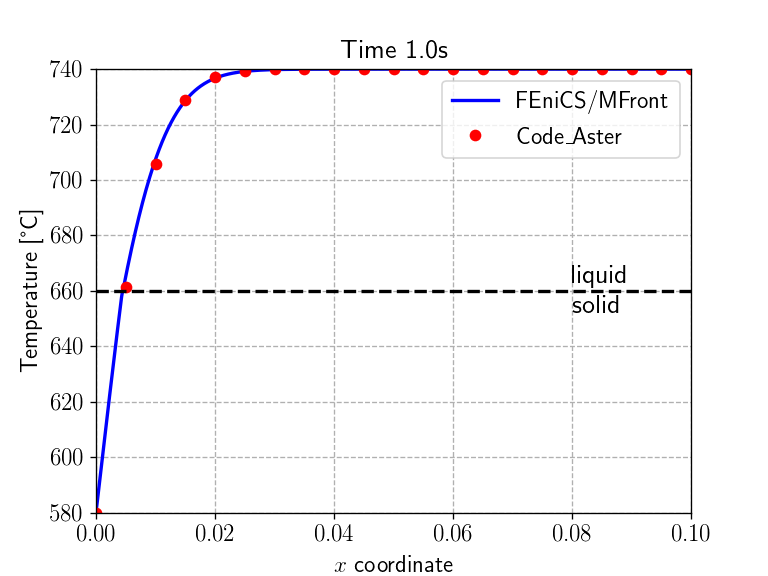<IPython.core.display.Javascript object>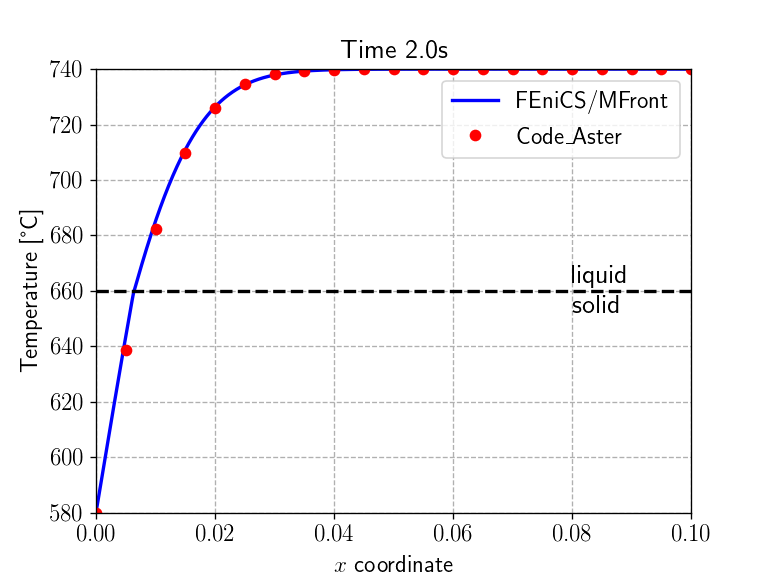<IPython.core.display.Javascript object>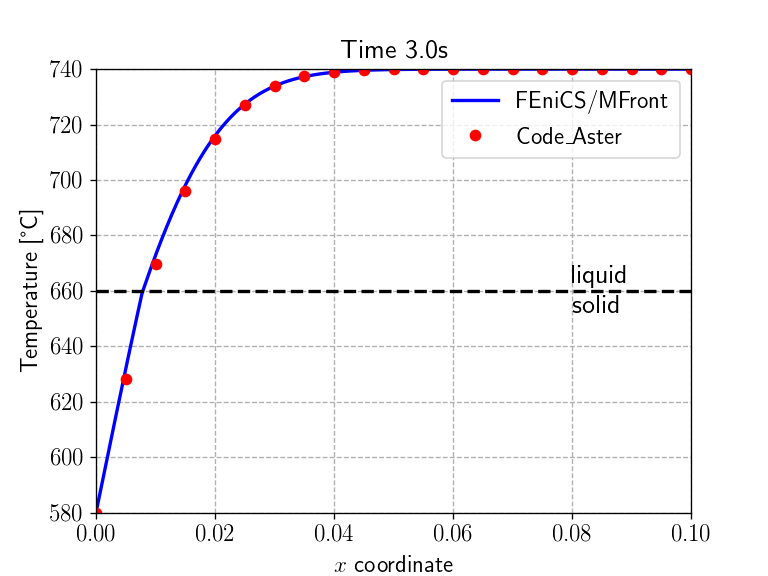<IPython.core.display.Javascript object>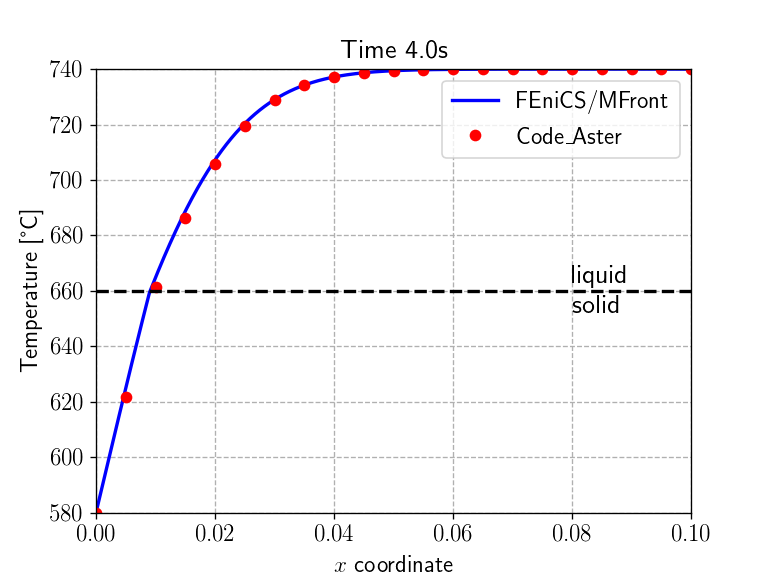<IPython.core.display.Javascript object>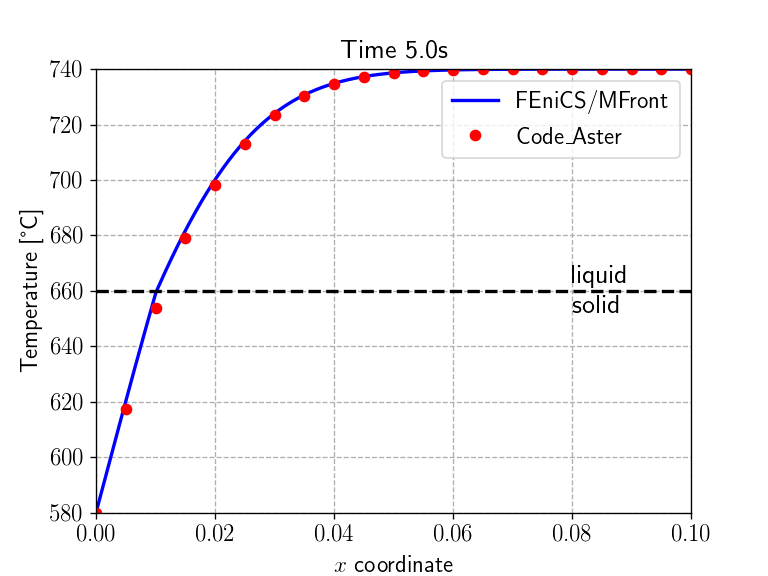<IPython.core.display.Javascript object>